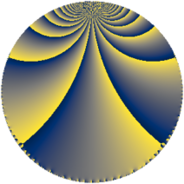# Properties

 Label 252.4.wLevel $252$ Weight $4$ Character orbit 252.w Rep. character $\chi_{252}(5,\cdot)$ Character field $\Q(\zeta_{6})$ Dimension $48$ Newform subspaces $1$ Sturm bound $192$ Trace bound $0$

# Learn more

## Defining parameters

 Level: $$N$$ $$=$$ $$252 = 2^{2} \cdot 3^{2} \cdot 7$$ Weight: $$k$$ $$=$$ $$4$$ Character orbit: $$[\chi]$$ $$=$$ 252.w (of order $$6$$ and degree $$2$$) Character conductor: $$\operatorname{cond}(\chi)$$ $$=$$ $$63$$ Character field: $$\Q(\zeta_{6})$$ Newform subspaces: $$1$$ Sturm bound: $$192$$ Trace bound: $$0$$

## Dimensions

The following table gives the dimensions of various subspaces of $$M_{4}(252, [\chi])$$.

Total New Old
Modular forms 300 48 252
Cusp forms 276 48 228
Eisenstein series 24 0 24

## Trace form

 $$48q + 6q^{7} + 12q^{9} + O(q^{10})$$ $$48q + 6q^{7} + 12q^{9} + 12q^{11} - 36q^{13} + 66q^{15} - 72q^{17} + 24q^{21} + 30q^{23} - 600q^{25} - 396q^{27} + 42q^{29} + 390q^{35} + 84q^{37} - 840q^{39} - 618q^{41} - 42q^{43} + 366q^{45} + 396q^{47} + 318q^{49} - 738q^{51} - 1620q^{53} + 492q^{57} + 1500q^{59} + 672q^{63} - 588q^{67} - 924q^{69} + 564q^{75} - 2472q^{77} + 1608q^{79} + 2592q^{81} - 360q^{85} + 2640q^{87} + 1722q^{89} + 540q^{91} + 660q^{93} - 792q^{97} - 54q^{99} + O(q^{100})$$

## Decomposition of $$S_{4}^{\mathrm{new}}(252, [\chi])$$ into newform subspaces

Label Dim. $$A$$ Field CM Traces $q$-expansion
$$a_2$$ $$a_3$$ $$a_5$$ $$a_7$$
252.4.w.a $$48$$ $$14.868$$ None $$0$$ $$0$$ $$0$$ $$6$$

## Decomposition of $$S_{4}^{\mathrm{old}}(252, [\chi])$$ into lower level spaces

$$S_{4}^{\mathrm{old}}(252, [\chi]) \cong$$ $$S_{4}^{\mathrm{new}}(63, [\chi])$$$$^{\oplus 3}$$$$\oplus$$$$S_{4}^{\mathrm{new}}(126, [\chi])$$$$^{\oplus 2}$$